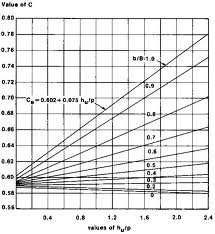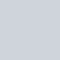# Drainage Co-efficientThe depth in centimeters of water drained off from a given area in 24 hours is called the drainage co-efficient.

D.C = Total water discharge in 24 hrs (m3)
______________________________
Total land area in sq. meters

Ditch capacity (Discharge rate) = Total quantity of water discharged in 24 hrs (cum)
________________________________________
60 X 60 X 24

Example:

A drainage canal discharge 0.2 cubic meters of water / sec and drains 250 hectares what will be drainage coefficient of this land?

Total water discharge in 24 hrs = 0.2 x 60 X 60 X 24
= 17280 m3

:. DC = 17280 / 250 X 104 = 0.0069m
= 0.69 cm =7 mm

Source